# February 2021## Trigonometric Ratios(Qns.& Ans) V.S.A.Q.’S

Trigonometric Ratios(Qns.& Ans) V.S.A.Q.’S designed by the ‘Basics in Maths‘ team.These notes to do help intermediate First-year Maths students.

Inter Maths – 1A two marks questions and solutions are very useful in I.P.E examinations.

## Trigonometric Ratios Up to Transformations

Question 1

Find the value of sin2(π/10) + sin2(4π/10) + sin2(6π/10) + sin2(9π/10)

Sol:

sin2(π/10) + sin2(4π/10) + sin2(6π/10) + sin2(9π/10)

= sin2(π/10) + sin2(π/2 – π/10) + sin2(π/2+ π/10) + sin2(π – π/10)

= sin2(π/10) + cos2(π/10) + cos2(π/10) + sin2(π/10)

= 1 + 1 = 2

Question 2

If sin θ = 4/5 and θ not in the first quadrant, find the value of cos θ

Sol:

Given sin θ = 4/5 and θ not in the first quadrant

⇒ θ in the second quadrant

⇒ cos θ < 0

cos2θ = 1 – sin2 θ

=1 – (4/5)2

= 1 – 16/25

∴cos θ   = – 3/5 (∵cos θ < 0)

Question 3

If 3sin θ + 4 cos θ = 5, then find the value of 4 sin θ – 3cos θ

Sol:

Given, 3sin θ + 4 cos θ = 5

let 4 sin θ – 3cos θ = x

(3sin θ + 4 cos θ )2 + (4 sin θ – 3cos θ)2 = 52 + x2

9 sin2 θ + 16 cos2 θ + 12 sin θ cos θ + 16 sin2 θ + 9 cos2 θ – 12sin θ cis θ = 25 + x2

25 sin2 θ + 25 cos2 θ = 25 + x2

25 = 25 + x2

⇒ x2 = 0

x = 0

∴ 4 sin θ – 3cos θ = 0

#### Question 4

If sec θ + tan θ =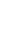, find the value of sin θ and determine the quadrant in which θ lies

Sol:

Given, sec θ + tan θ =  ———— (1)

We know that sec2 θ – tan2 θ = 1

⇒ (sec θ + tan θ) (sec θ – tan θ) = 1

(1) + (2)

(1) – (2)

Since sec θ positive and tan θ is negative θ lies in the 4th quadrant.

Question 5

Prove that cot (π/16). cot (2π/16). cot (3π/16).… cot (7π/16) = 1

Sol:

cot (π/16). cot (2π/16). cot (3π/16).… cot (7π/16)

= cot (π/16). cot (2π/16). cot (3π/16). cot (4π/16). cot (5π/16) cot (6π/16) cot (7π/16)

= cot (π/16). cot (2π/16). cot (3π/16). cot (π/4). cot (π/2 – 3π/16) cot (π/2 – 2π/16) cot (π/2 – π/16)

= cot (π/16). cot (2π/16). cot (3π/16). cot (π/4). tan (3π/16) tan (2π/16) tan (π/16)

= [cot (π/16). tan (π/16)] [cot (2π/16). tan (2π/16)] [cot (3π/16). tan (3π/16]. cot (π/4)

= 1.1.1.1

=1

Question 6

If cos θ + sin θ =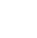cos θ, then prove that cos θ – sin θ =  sin θ

Sol:

Question 7

Find the value of 2(sin6 θ + cos6 θ) – 3 (sin4 θ + cos4 θ)

Sol:

2(sin6 θ + cos6 θ) – 3 (sin4 θ + cos4 θ)

= 2[(sin2 θ)3 + (cos2 θ)3] – 3[(sin2 θ)2 + (cos2)2

= 2[(sin2 θ + cos2 θ)3 – 3 sin2 θ cos2 θ (sin2 θ + cos2 θ)] – 3[(sin2 θ + cos2 θ)2 – 2 sin2 θ cos2 θ]

= 2[1 – 3 sin2 θ cos2 θ] – 3 [1 – 2 sin2 θ cos2 θ]

= 2 – 6 sin2 θ cos2 θ – 3 + 6 sin2 θ cos2 θ

= – 1

##### Question 8

Sol:

Given tan 200 = λ

###### Question 9

If sin α + cosec α = 2, find the value of sinn α + cosecn α, n∈ Z

Sol:

Given sin α + cosec α = 2

⇒ sin α + 1/ sin α = 2

sin2 α + 1= 2 sin α

sin2 α – 2 sin α + 1= 0

(sin α – 1 )2 = 0

⇒ sin α – 1 = 0

sin α = 1 ⇒ cosec α = 1

sinn α + cosecn α = (1)n + (1)n =1 + 1 =2

∴ sinn α + cosecn α = 2

Question 10

Sol:Question 11

Find the value of sin 3300. cos 1200 + cos 2100. Sin 3000

Sol:

sin 3300. cos 1200 + cos 2100. Sin 3000

=sin (3600 – 300). cos (1800 – 600) + cos (1800 + 300). sin (3600 – 600)

= (– sin 300). (– cos 600) + (– cos300). (– sin600)

= sin 300.  cos 600 + cos300.  Sin600

= sin (600 + 300) = sin 900

=1

Question 12

Sol:

= cos4 α + 2 cos2 α (1 – cos2 α)

= (cos2 α)2 + 2 (1 – sin2 α) (sin2 α)

= (1 – sin2 α)2 + 2 sin2 α – 2sin4 α

= 1 + sin4 α – 2 sin2 α + 2 sin2 α – 2sin4 α

= 1 – sin4 α

Question 13

Eliminate θ from x = a cos3 θ and y = b sin3 θ

Sol:

Given x = a cos3 θ and y = b sin3 θ

cos3 θ = x/a and sin3 θ = y/b

cos θ = (x/a)1/3 and sin θ = (y/b)1/3

we know that sin2 θ + cos2 θ = 1

⇒ [(y/b)1/3]2 + [(x/a)1/3]2 = 1

(x/a)2/3 + (y/b)2/3 = 1

Question 14

Find the period of the following functions

Sol:

(i) f(x) = tan 5x

period of given function is = LCM (8, 6) = 24

(iv) f(x) = tan (x + 4x + 9x +…. + n2x)

f(x) = tan (x + 4x + 9x +…. + n2x)

= tan (1 + 4 + 9 + … + n2) x

Question 15

Sol:

We know that sin2 A – sin2B = sin (A +B) sin (A – B)

⇒ sin2(52 ½)0 – sin2 (22 ½)0

= sin (52 ½+ 22 ½) sin (52 ½ – 22 ½)

= sin 750 sin 300

Question 16

Prove that tan 700 – tan200 = 2 tan 500

Sol:

500 = 700 – 200

Tan 500 = tan (700 – 200)

⇒ tan 700 – tan 200 = tan 500 (1 + tan700 tan 200)

tan 700 – tan 200 = tan 500 [1 + tan700 cot (900 – 200)]

tan 700 – tan 200 = tan 500 [1 + tan700 cot 700]

tan 700 – tan 200 = tan 500 [1 + 1]

∴ tan 700 – tan200 = 2 tan 500

Question 17

Sol:tan α = 1/3                                                          tan β = ½

tan (α + β) = 1

#### Question 18

Sol:

Question 19

Sol:

(on dividing numerator and denominator by cos 90)

= tan (450 + 90)

= tan 540

= tan (900 – 360)

= cot 360

Question 20

Show that cos 420 + cos 780 + cos 1620 = 0

Sol:

cos 420 + cos 780 + cos 1620

= cos (600 – 180) + cos (600 + 180) + cos (1800 – 180)

=cos 600 cos180 + sin 600 sin 180 + cos 600 cos 180 – sin 600 sin 180 – cos 180

= 2 cos 600 cos 180 – cos 180

= 2 (1/2) cos 180 – cos 180

= cos 180 – cos 180

= 0

Question 21

Sol:

= 2(cos 300 sin θ + sin 300 cos θ)

= 2 sin (θ + 300)

Question 22

Find the maximum and minimum value of the following functions

(i) 3 sin x –4 cos x

a= 3, b = –4 and c = 0

= 5

∴ minimum value = –5 and maximum value = 5

(ii) cos (x + ) + 2  sin (x + ) – 3

a= 1, b = 2  and c = – 3∴ minimum value = –6 and maximum value = 0

Question 23

#### Find the range of the function f(x) = 7 cos x – 24sin x + 5

Sol:

Given f(x) = 7 cos x – 24sin x + 5

a= 7, b = –24 and c = 5

∴ Range = [–20, 30]

Question 24

Prove that sin2α + cos2 (α + β) + 2 sin α sin β cos (α + β) is independent of α

Sol:

sin2α + cos2 (α + β) + 2 sin α sin β cos (α + β)

= sin2α + cos (α + β) [ cos (α + β) +2 sin α sin β]

= sin2α + cos (α + β) [ cos α cos β – sin α sin β +2 sin α sin β]

=sin2α + cos (α + β) [ cos α cos β + sin α sin β]

=sin2α + cos (α + β) cos (α –β)

= sin2 α + cos2 α – sin2 β

=1 – sin2 β

= cos2 β

Question 25

Sol:

= tan θ

Question 26

Sol:

⟹ 0 < 2x < π/2 (∵ x is in first quadrant)

⟹ 0 < x < π/4

Question 27

Sol:

π < θ < 3π/2 ⟹ π/2 < θ/2 < 3π/4

tan θ/2 < 0

= – 2

Question 28

If A is not an integral multiple of π/2, prove that cot A – tan A = 2 cot 2A.

Sol:

= 2 cot 2A

Question 29

Evaluate 6 sin 200 – 8sin3 200

Sol:

6 sin 200 – 8sin3 200 = 2 (3 sin 200 – 4sin3 200)

= 2 sin 3(200)

= 2 sin 600

Question 30

Express cos6 A + sin6 A in terms of sin 2A.

Sol:

cos6 A + sin6 A

= (sin2 A)3 + (cos2 A)3

= (sin2 A + cos2 A)3 – 3 sin2 A cos2 A (sin2 A + cos2 A)

= 1 – 3 sin2 A cos2 A

=1 – ¾ (4 sin2 A cos2 A)

= 1 – ¾ sin22 A

Question 31

Sol:=2 cos (θ/2)

Question 32

Find the extreme values of cos 2x + cos2x

Sol:

cos 2x + cos2x = 2cos2 x– 1 + cos2 x

=3cos2 x – 1

We know that – 1 ≤ cos x ≤ 1

⟹ 0 ≤ cos2 x ≤ 1

3×0 ≤ 3×cos2 x ≤ 3×1

0– 1 ≤3 cos2 x – 1≤ 3– 1

– 1≤3 cos2 x – 1≤ 2

Minimum value = – 1

Maximum value = 2

Question 33

Sol: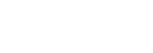= 4

Question 34

Sol:

sin 780 + cos 1320 = sin 780 + cos (900 + 420)

= sin 780 – sin 420

= 2 cos 600 sin 180

=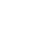Question 35

Find the value of sin 340 + cos 640 – cos40

Sol:

sin 340 + cos 640 – cos40

= sin 340 – 2sin 340 sin 300

= sin 340 – 2 sin 340 (1/2)

=sin 340 – sin 340

=0

##### Question 36

Sol:

4(cos 660 + sin 840)

=4(cos 660 + sin (900 – 60)

=4(cos 660 + cos (60)

=8 cos 360 cos 300

=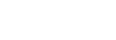Question 35

Prove that (tan θ + cot θ)2 = sec2 θ + cosec2 θ = sec2 θ. cosec2 θ

Sol:

= sec θ. cosec θ

(tan θ + cot θ)2   = sec2 θ. cosec2 θ

= sec2 θ. cosec2 θ

##### Visit my Youtube Channel: Click on Below Logo## Product of Vectors (Qns.& Ans) V.S.A.Q.’S

Product of Vectors (Qns.& Ans) V.S.A.Q.’S; These solutions were designed by the ‘Basics in Maths‘ team. These notes to do help intermediate First-year Maths students.

Inter Maths – 1A two marks questions and solutions are very useful in IPE examinations.

### Product Of Vectors

Question 1

If a = 6i +2 j +3 k, b = 2i – 9 j+ 6k, then find the angle between the vectors a and b

Sol:

Given vectors are a = 6i +2 j +3 k, b = 2i – 9 j+ 6k

a .b = (6i +2 j +3 k). (2i – 9 j+ 6k) = 6(2) + 2 (– 9) + 3(6)

= 12 – 18 + 18 = 12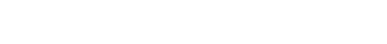= 7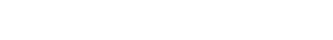= 11

Question 2

If a = i +2 j –3 k, b = 3ij+ 2k, then show that a + b and ab are perpendicular to each other.

Sol:

Given vectors are a = i +2 j –3 k, b = 3ij+ 2k

a + b = (i +2 j –3 k) + (3ij+ 2k) = 4i + jk

ab = (i +2 j –3 k) – (3ij+ 2k) = –2i +3 j – 5k

(a + b). (ab) = (4i + jk). (–2i +3 j – 5k)

= – 8 + 3 + 5

= 0

a + b and ab is perpendicular to each other.

### Question 3

If a and b be non-zero, non-collinear vectors. If , then find the angle between a and b

Sol:

Squaring on both sides

(a + b) (a + b) = (a – b) (a – b)

a2 + 2 a. b + b2 = a2 – 2 a.b + b2

⟹ 4 a.b = 0

a.b = 0

∴ the angle between a and b  is 900

### Question 4

Sol:

(11)2 2 ×11×23 cos θ + (23)2 = 900

121 506 cos θ + 529   = 900

650 506 cos θ = 900

= (11)2 + 2 ×11×23 cos θ + (23)2

= 121 + 2 ×11×23 ×  + 529

= 400

### Question 5

If a = ijk and b = 2i – 3j + k, then find the projection vector of b on a and its magnitude.

Sol:

Given vectors are a = ijk and b = 2i – 3j + k

a.b = (ij k). (2i – 3j + k) = 2 + 3 – 1 = 4

### Question 6

If the vectors λ i – 3j + 5k and 2λ i – λ jk are perpendicular to each other, then find λ

Sol:

let a = λ i – 3j + 5k, b = 2λ i – λ jk

Given, that a and b are perpendicular to each other

a.b = 0

i – 3j + 5k). (2λ i – λ jk) = 0

2 λ2 + 3 λ – 5 = 0

2 λ2 + 5 λ – 2 λ – 5 = 0

λ (2 λ + 5) – 1 (2 λ + 5) = 0

(2 λ + 5) ((λ – 1) = 0

λ = 1 or λ = -5/2

### Question 7

Find the Cartesian equation of the plane passing through the point (– 2, 1, 3) and perpendicular to the vector 3i + j + 5k

Sol:

let P (x, y, z) be any point on the plane

⟹ OP = xi + yj + zk

OA = – 2i +j +3k

AP = OPOA = (xi + yj + zk) – (– 2i +j +3k)

AP = (x + 2) i + (y – 1) j + (z – 3) k

AP is perpendicular to the vector 3i + j + 5k

⟹ 3 (x + 2) + (y – 1) + 5(z – 3) = 0

⟹ 3x + 6 + y – 1 + 5z – 15 = 0

∴   3x + y + 5z – 10 = 0 is the required Cartesian equation of the plane

### Question 8

Find the angle between the planes 2x – 3y – 6z = 5 and 6x + 2y – 9z = 4

Sol:

Given plane equations are: 2x – 3y – 6z = 5,6x + 2y – 9z = 4

Vector equations of the above planes are: r. (2i – 3j – 6k) = 5 and r. (6i + 2j – 9k) = 4

⟹ n1 = 2i – 3j – 6k and n2 = 6i + 2j – 9k

If θ is the angle between the planes r. n1 = d1 and r. n2 = d2, then

### Question 9

a = 2ij + k, b = i – 3j – 5k. Find the vector c such that a, b, and c form the sides of a triangle.

Sol:

Given a = 2ij + k, b = i – 3j – 5k

If a, b, and c form the sides of a triangle, then a + b + c = 0

⟹ a + b = – c

c = – (a + b)

= – [(2ij + k) +( i – 3j – 5k)]

= – (3i –4 j –4k)

∴ c = – 3i +4 j + 4k

### Question 10

Find the equation of the plane through the point (3, –2, 1) and perpendicular to the vector (4, 7, –4).

Sol:

Let a = 3i – 2j + k and n = 4i + 7j – 4k

The equation of the plane passing through point A(a) and perpendicular to the vector n is (ra). n = 0

⟹ [r – (3i – 2j + k)]. (4i + 7j – 4k) = 0

⟹ r. (4i + 7j – 4k)– [(3i – 2j + k). (4i + 7j – 4k)] = 0

r. (4i + 7j – 4k)– (12 – 14 – 4) = 0

r. (4i + 7j – 4k)– 6 = 0

r. (4i + 7j – 4k) = 6

### Question 11

Find the unit vector parallel to the XOY-plane and perpendicular to the vector 4i – 3j + k

Sol:

The vector which is parallel to the XOY-plane is of the form xi + yj

The vector which is parallel to the XOY-plane and perpendicular to 4i – 3j + k

is 3i + 4j

∴ The unit vector parallel to the XOY-plane and perpendicular to the vector 4i – 3j + k =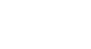### Question 12

Sol:

Given, a + b + c = 0,  = 3,  = 5 and    = 7

a + b = – c

32 + 52 + 2  cos θ = 72

9 + 25 + 2.3.5 cos θ = 49

34 + 30cos θ = 49

30cos θ = 49 – 34

30cos θ = 15

cos θ = 15/30 = 1/2

∴ θ = π/3

### Question 13

If a = 2i – 3j + 5k, b = – i + 4j + 2k, then find a × b and unit vector perpendicular to both a and b

Sol:

Given, a = 2i – 3j + 5k, b = – i + 4j + 2k

= i (–6 – 20) – j (4 + 5) + k (8 – 3)

= –26i – 9j + 5k

### Question 14

If a = i + j + 2k and b = 3i + 5jk are two sides of a triangle, then find its area.

Sol:

Given, a = i + 2j + 3k and b = 3i + 5jk

If a, b are two sides of a triangle, then area of the triangle =

= i (–2 – 15) – j (–1 – 9) + k (5 – 6)

= –17i + 10 j k

### Question 15

Find the area of the parallelogram for which the vectors a = 2i – 3j and b = 3ik are adjacent sides.

Sol:

Given, a = 2i – 3j and b = 3ik are adjacent sides of a parallelogram

The area of the parallelogram whose vectors a , b are adjacent sides =

= i (3 – 0) – j (–2 – 0) + k (0 + 9)

=3 i +2 j +9 k

### Question 16

Let a, b be two non-collinear unit vectors. If α = a – (a . b) b and β = a × b, then show that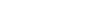Sol:

= 1 – cos2 θ

= sin2 θ

= 1 + cos2 θ – 2cos2 θ

= 1– cos2 θ

= sin2 θ

### Question 17

Sol:

Let a = xi + yj + zk

= i ( 0 – 0) – j (0 – z) + k (0 – y)

= – yk + zj

= 2(x2 + y2 +z2)

### Question 18

Sol:

= 2 × 3 sin

= 2 × 3×1/2

= 3

### Question 19

If 4i + j + pk is parallel to the vector i + 2j + 3k, then find p.

Sol:

Given 4i +  j + pk is parallel to the vector i + 2j + 3k

⇒ p = 12

### Question 20

Compute a× (b + c) + b× (c + a) + c× (a + b)

Sol:

a× (b + c) + b× (c + a) + c× (a + b)

= a× b + a× c + b× c + b× a + c × a + c × b

= a× b + a× c + b× ca × b a × c b ×c

= 0

### Question 21

Compute 2j× (3i – 4k) + (i + 2j) × k

Sol:

2j× (3i – 4k) + (i + 2j) × k

= 6(j × i) – 8(j × k) + (i × k) + 2(j × k)

= 6k – 8i – j + 2i

= 6i –j 6 j

##### Visit my Youtube Channel: Click on Below Logo## Addition of Vectors (Qns.& Ans) V.S.A.Q.’S

These solutions were designed by the ‘Basics in Maths’ team. These notes to do help intermediate First-year Maths students.

Inter Maths – 1A two marks questions and solutions are very useful in IPE examinations.

#### QUESTION 1#### QUESTION 2

Find a vector in the direction of a where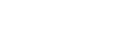that has a magnitude of 7 units.

The vector having the magnitude 7 and in the direction of  is

#### QUESTION 3

Find the unit vector in the direction of the sum of the vectors, a = 2i + 2j – 5k and b = 2i + j + 3k

Sol Given vectors are a = 2i + 2j – 5k and b = 2i + j + 3k

a + b = (2i + 2j – 5k) + (2i + j + 3k) = 4i + 3j – 2k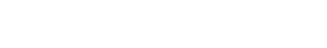##### QUESTION 4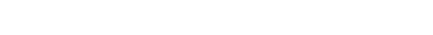QUESTION 5

Show that the points whose position vectors are – 2a + 3b + 5c, a + 2b + 3c, 7 ac are collinear when a, b, c are non-collinear vectors

Sol: Let OA = – 2a + 3b + 5c, OB = a + 2b + 3c, OC = 7 acA

B = OB – OA = a + 2b + 3c (– 2a + 3b + 5c)

AB = 3a b – 2c

AC = OC – OA = 7 ac(– 2a + 3b + 5c)

AC = 9a – 3b – 6c = 3(3a b – 2c)

AC = 3 AB

A, B and C are collinear

##### QUESTION 6

ABCD is a parallelogram if L and M are middle points of BC and CD. Then find (i) AL and AM in terms of AB and AD (ii) 𝛌, if AM = 𝛌 AD – LM

Sol: Given, ABCD is a parallelogram and L and M are middle points of BC and CD(i) Take A as the origin

M is the midpoint of CD

= AD + ½ AB (∵ AB = DC)

L is the midpoint of BC

(ii) AM = 𝛌 AD – LM

𝛌 = 3/2

#### QUESTION 7

Sol: OA = a, OB = b, OC = c and OD = dD is the midpoint of BC

G divides median AD in the ratio 2: 1

##### QUESTION 8Equating like vectors

2 = 4 λ; 5 = m λ; 1 = n λ

∴ m = 10, n = 2

#### QUESTION 9

##### QUESTION 10

If the vectors – 3i + 4j + λk and μi + 8j + 6k. are collinear vectors, then find λ and μ.

Sol: let a = – 3i + 4j + λk, b = μi + 8j + 6k

⟹   a = tb

– 3i + 4j + λk = t (μi + 8j + 6k)

– 3i + 4j + λk = μt i + 8t j + 6t k

Equating like vectors

– 3 = μt; 4 = 8t, λ = 6t

4 = 8t

∴ μ=– 6, λ = 3

##### QUESTION 11

ABCD is a pentagon. If the sum of the vectors AB, AE, BC, DC, ED and AC is 𝛌 AC then find the value of 𝛌

Sol: Given, ABCD is a pentagon

AB + AE + BC + + DC + ED + AC = 𝛌 AC

(AB + BC) + (AE + DC + ED) + AC = 𝛌 AC

AC + AC + AC = 𝛌 AC

3 AC = 𝛌 AC

𝛌 = 3

#### QUESTION 12

If the position vectors of the points A, B and C are – 2i + jk and –4i + 2j + 2k and 6i – 3j – 13k respectively and AB = 𝛌 AC, then find the value of 𝛌

Sol: Given, OA = – 2i + jk , OB = –4i + 2j + 2k and OC  = 6i – 3j – 13k

AB = OB – OA = –4i + 2j + 2k – (– 2i + jk)

= –4i + 2j + 2k +2ij + k

= –2i + j + 3k

AC = OC – OA = 6i – 3j – 13k – (– 2i + jk)

= 6i – 3j – 13k +2ij + k

= 8i –4 j –12k

= – 4 (2i + j + 3k)

AC = – 4 AB

Given AB = 𝛌 AC

𝛌 = – 1/4

#### QUESTION 13

If OA = i + j +k, AB = 3i – 2j + k, BC = i + 2j – 2k, CD = 2i + j +3k then find the vector OD

Sol: Given OA = i + j +k, AB = 3i – 2j + k, BC = i + 2j – 2k, CD = 2i + j +3k

OD = OA + AB + BC + CD

= i + j +k + 3i – 2j + k + i + 2j – 2k + 2i + j +3k

= 7i + 2j +4k

#### QUESTION 14

Let a = 2i +4 j –5 k, b = i + j+ k, c = j +2 k, then find the unit vector in the opposite direction of a + b + c

Sol:  Given, a = 2i +4 j –5 k, b = i + j+ k, c = j +2 k

a + b + c = 2i +4 j –5 k + i + j+ k + j +2 k

= 3i +6j –2k

##### QUESTION 15

Is the triangle formed by the vectors 3i +5j +2k, 2i –3j –5k, 5i – 2j +3k

Sol: Let a =3i +5j +2k, b = 2i –3j –5k, c = 5i – 2j +3k∴ Given vectors form an equilateral triangle.

##### QUESTION 16

Using the vector equation of the straight line passing through two points, prove that the points whose position vectors are a, b,  (3a – 2b) are collinear.

Sol: the vector equation of the straight line passing through two points a, b is

r = (1 – t) a+ t b

3a – 2b = (1 – t) a+ t b

Equating like vectors

1 – t = 3 and t = – 2

∴ Given points are collinear.

###### QUESTION 17

OABC is a parallelogram If OA = a and OC = c, then find the vector equation of the side BC

Sol: Given, OABC is a parallelogram and OA = a, OC = cThe vector equation of BC is a line which is passing through C(c) and parallel to OA

⟹ the vector equation of BC is r = c + t a

##### QUESTION 18

If a, b, c are the position vectors of the vertices A, B, and C respectively of triangle ABC, then find the vector equation of the median through the vertex A

Sol: Given OA = a, OB = b, OC = cD is mid of BC

#### QUESTION 19

Find the vector equation of the line passing through the point 2i +3j +k and parallel to the vector 4i – 2j + 3k

Sol: Let a =2i +3j +k, b = 4i – 2j + 3k

The vector equation of the line passing through a and parallel to the vector b is r = a + tb

r = 2i +3j +k + t (4i – 2j + 3k)

= (2 + 4t) i + (3 – 2t) j + (1 + 3t) k

##### QUESTION 20

Find the vector equation of the plane passing through the points i – 2j + 5k, 2j –k and – 3i + 5j

Sol: The vector equation of the line passing through a, b and cis r = (1 – t – s) a + tb + sc

r = (1 – t – s) (i – 2j + 5k) + t (2j –k) + s (– 3i + 5j)

= (1 – t – 4s) i + (– 2 – 3t + 7s) j + (5 – 6t – 5s) k

##### Visit my Youtube Channel: Click on Below Logo## The Plane (2m Questions & Solutions) || V.S.A.Q’S||

The Plane: These solutions were designed by the ‘Basics in Maths’ team. These notes to do help intermediate First-year Maths students.
Inter Maths – 1B two mark questions and solutions are very useful in IPE examinations.
These notes cover all the topics covered in the intermediate First-year Maths syllabus and include plenty of solutions to help you solve all the major types of Math problems asked in the IPE examinations.

### The Plane

#### Question 1

Find the equation of the plane if the foot of the perpendicular from the origin to the plane is (2, 3, – 5).

Sol:

The plane passes through A and is perpendicular to OA, then the line segment OA is normal to the plane.

Dr’s of OA = (2, 3, – 5)

The equation of the plane passing through the point (x1, y1, z1) and dr’s (a, b, c) is

a(x – x1) + b (y – y1) + c (z – z1) = 0

⟹ 2(x – 2) + 3 (y – 3) – 5 (z + 5) = 0

2x – 4 + 3y – 9 – 5z – 25 = 0

2x + 3y – 5z – 38 = 0

#### Question 2

Find the equation of the plane passing through the points (0, – 1, – 1), (4, 5, 1) and (3, 9, 4)

Sol:

The equation of the plane passing through the points (x1, y1, z1) (x2, y2, z2) (x3, y3, z3) isThe plane passing through the points (0, – 1, – 1), (4, 5, 1) and (3, 9, 4) is

x (30 – 20) – (y + 1) (20 – 6) + (z + 1) (40 – 18) = 0

x (10) – (y + 1) (14) + (z + 1) (22) = 0

10x – 14y – 14 + 22z + 22 = 0

10x – 14y + 22z + 8 = 0

2(5x – 7y + 11z + 4) = 0

∴ the equation of the plane is 5x – 7y + 11z + 4 = 0

##### Question 3

Find the equation to the plane parallel to the ZX-plane and passing through (0, 4, 4).

Sol:

Equation of ZX-plane is y = 0

The equation of the plane parallel to the ZX-plane is y = k

But it is passing through (0, 4, 4)

⟹ y = 4

###### Question 4

Find the equation to the plane passing through the point (α, β, γ) and parallel to the plane axe + by + cz + d = 0.

Sol:

The equation of the plane parallel to the plane ax + by + cz + d = 0 is ax + by + cz + k = 0

But it is passing through the point (α, β, γ)

a α + b β + c γ + k = 0

⟹ k = – a α – b β – c γ

The equation of the plane is ax + by + cz – a α – b β – c γ = 0

⟹ a(x – α) + b (y – β)+ c (z – γ) = 0

#### Question 5

Find the angle between the planes 2x – y + z = 6 and x + y + 2z = 7.

Sol: If θ is the angle between the planes a1 x + b1 y + c1 z + d1 = 0 and a2x + b2 y + c2 z + d2 = 0, then cos θ =Cos θ = cos 600

θ = 600 =

##### Question 6

Reduce the equation x + 2y – 2z – 9 = 0 to the normal form and hence find the dc’s of the normal to the plane.

Sol: Given plane is x + 2y – 2z – 9 = 0

x + 2y – 2z = 9

##### Question 7

Suppose a plane makes intercepts 2, 3, 4 on X, Y, Z axes respectively. Find the equation of the plane in the intercept form.

Sol: Given a = 2, b = 3, c = 4

#### Question 8

Express x – 3y + 2z = 9 in the intercept form

Sol: Given plane is x – 3y + 2z = 9

a = 9, b = – 3, c = 9/2

#### Question 9

Find the direction cosine of the normal to the plane x + 2y + 2z – 4 = 0.

Sol: Given plane is x + 2y + 2z – 4 = 0

We know that Dr’s of the normal to the plane ax + by + cz + d = 0 are (a, b, c)

⟹ dr’s of the normal to the plane x + 2y + 2z – 4 = 0 are (1, 2, 2)

##### Question 10

Find the midpoint of the line joining the points (1, 2, 3) and (–2, 4, 2)

Sol: Given points are A (1, 2, 3), B (–2, 4, 2)

##### Visit my Youtube Channel: Click on Below Logo## 3D Coordinates (Q’s & Ans) || V.S.A.Q’S||

These solutions were designed by the ‘Basics in Maths‘ team. These notes to do help intermediate First-year Maths students.

Inter Maths – 1B two mark questions and solutions are very useful in IPE examinations.

### 3D Coordinates

1. Show that the points A (– 4, 9, 6), B (– 1, 6, 6), and C (0, 7, 10) form a right-angled isosceles triangle.

Sol:

Distance between two points P (x1, y1, z1) and Q (x2, y2, z2) is PQ =∴ The points A (– 4, 9, 6), B (– 1, 6, 6) and C (0, 7, 10) form a right-angled isosceles triangle.

2. Show that the locus of the point whose distance from Y – axis is thrice its distance from (1, 2, – 1) is 8x 2 + 9y2 + 8 z2 – 18x – 36y + 18z + 54 = 0

Sol:

Let P (x, y, z) be the locus of the point

A (0, y, 0) be any point on Y – axis

B = (1, 2, – 1)

Condition is PA = 3PB

PA2 = (3PB)2

PA2= 9 PB

⟹ x2 + z2 = 9[(x – 1)2 + (y – 2)2 + (z + 1)2]

x2 + z2 = 9[x2 – 2x + 1 + y2 – 4y + 4 + z2 + 2z + 1]

x2 + z2 = 9x2 – 18x + 9 + 9y2 – 36y + 36 +9 z2 + 18z + 9

∴ 8x 2 + 9y2 + 8 z2 – 18x – 36y + 18z + 54 = 0

3. A, B, and C are three points on OX, OY, and OZ respectively, at distances a, b, c (a≠0, b≠0, c≠0) from the origin ‘O’. Find the coordinate of the point which is equidistant from A, B, C, and O

Sol:

Let P (x, y, z) be the required point

O = (0, 0, 0)   A = (a, 0, 0), B = (0, b, 0), C = (0, 0, c)

Given, AP = BP = CP = OP

AP = OP

⟹   AP2 =OP2

(x – a )2 + y2 + z2 = x2 + y2 + z2

x2 + a2 – 2ax + y2 + z2 = x2 + y2 + z2

a2 – 2ax = 0

a (a – 2x) = 0

a – 2x = 0 (∵ a≠0)

a = 2x ⟹ a/2

Similarly, y = b/2 and z = c/2

∴ P = (a/2, b/2, c/2)

4. Show that the points A (3, – 2, 4), B (1, 1, 1) and C (– 1, 4, – 2) are collinear

Sol:

Given points are A (3, – 2, 4), B (1, 1, 1), and C (– 1, 4, – 2)5.Find x if the distance between (5, – 1, 7), (x, 5, 1) is 9 units.

Sol:

Let A = (5, – 1, 7), B = (x, 5, 1)

Given AB = 9

⟹ AB2 = 81

(5 – x)2 + (– 1 – 5)2 + (7 – 1)2 = 81

(5 – x)2 + 36 + 36 = 81

(5 – x)2 + 72 = 81

(5 – x)2 = 81 – 72 = 9

(5 – x) = ± 3

5 – x = 3 or 5 – x = – 3

5 – 3 = x or 5 + 3 = x

x = 2 or x = 8

6.If the point (1, 2, 3) is changed to point (2, 3, 1) through the translation of axes. Find a new origin.

Sol:

Given (x, y, z) = (1, 2, 3) and (X, Y, Z) = (2, 3, 1)

x = X + h, y = Y + k, z = Z + l

h = x – X, k = y – Y, l = z – Z

h = 1 – 2, k = 2 – 3, l = 3 – 1

h = – 1, k = – 1, l = 2

New origin is (– 1, – 1, 2)

7.By section formula, find the point which divides the line joining the points (2, – 3, 1) and (3, 4, – 5) in the ratio 1 : 3.

Sol:

If a point P divides the line segment joining the points (x1, y1, z1), (x2, y2, z2) in the ratio, thenLet P divides the line joining the points (2, – 3, 1) and (3, 4, – 5) in the ratio 1 : 38.Find the ratio in which the line joining two points (7, 0, – 1) and (– 2, 3, 5) is divided by the point (1,2,3).

Sol:

Let A = (7, 0, – 1), B = (– 2, 3, 5) and P = (1,2,3)

Suppose P divides AB in the ratio k : 19.Using section formula, verify whether the points A (2, –4, 3), B (–4, 5, 6), C (4, –7, 2) are collinear or not.

Sol:

Given Points are A (2, –4, 3), B (–4, 5, 6), C (4, –7, 2)

Let C divides AB in the ratio k : 12 – 4k = 4 (k + 1)

2 – 4k = 4k + 4

– 4k– 4k = 4 – 2

– 8k = 2

K = -1/4

C divides AB in the Ratio 1 : 4 externally

∴ A, B, C are collinear

10.Find the centroid of the triangle whose vertices are (5, 4, 6), (1, –1, 3) and (4, 3, 2)

Sol:

The centroid of the triangle whose vertices are (x1, y1, z1), (x2, y2, z2) and (x3, y3, z3) is11. Find the centroid of the tetrahedron whose vertices are (2, 3, –4), (–3, 3, –2), (–1, 4, 2) and (3, 5, 1)

Sol:

The centroid of the tetrahedron whose vertices are (x1, y1, z1), (x2, y2, z2) (x3, y3, z3), and (x4, y4, z4) is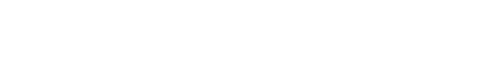the centroid of the tetrahedron whose vertices are (2, 3, –4), (–3, 3, –2), (–1, 4, 2) and (3, 5, 1) is12. Find the ratio in which the YZ-plane divides the line joining A(2, 4, 5) and B (3, 5, – 4)

Sol:

Let P be any point on the YZ-plane

P = (0, y, z)

Let P divides AB int eh ratio k:1YZ-plane divides AB in the ratio – 2:3

13. Find the fourth vertex of the parallelogram whose consecutive vertices are (2, 4, – 1), (3, 6, – 1) and (4, 5, 1).

Sol:

let A = (2, 4, – 1), B = (3, 6, – 1), C = (4, 5, 1) and D = (x, y, z)

ABCD is a parallelogram

Midpoint of AC = Midpoint of BD⟹ 3 + x = 6, 6 + y = 9, – 1 + z = 0

x = 3, y = 3, z = 1

∴ The fourth vertex D = (3, 3, 1)

14. A (5, 4, 6), B (1, –1, 3), and C (3, 3, 1) are three points. Find the coordinates of the point at which the bisector of ∠BAC meets the side BC.

Sol:

We know that the bisector of ∠BAC divides BC in the ratio AB: ACIf D is a point where the bisector of ∠BAC meets BC

⟹ D divides BC in the ratio 5:315. If M (α, β, γ) is the midpoint of the line joining the points (x1, y1, z1) and B, then find B

Sol:

Let B (x, y, z) be the required point

M is the midpoint of AB

⟹ (α, β, γ) =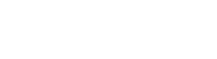⟹ 2 α = x + x1; 2 β = y + y1; 2 γ = z + z1

x =2 α – x1; x =2 β – y1; x =2 γ – z1

∴ B = (2 α – x1, 2 β – y1, 2 γ –)

16. If H, G, S, and I respectively denote the orthocenter, centroid, circumcenter, and incentre of a triangle formed by the points (1, 2, 3), (2, 3, 1) and (3, 1, 2), then find H, G, S, I.

Sol:

Let A = (1, 2, 3), B= (2, 3, 1), C = (3, 1, 2)⟹ ∆ ABC is an equilateral triangle

We know that, in an equilateral triangle orthocenter, centroid, circumcenter, and incentre are the same

Centroid G =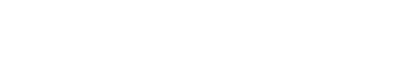= (2, 2, 2)

∴ H = (2, 2, 2), S = (2, 2, 2), I = (2, 2, 2)

17. Find the incentre of the triangle formed by the points (0, 0, 0), (3, 0, 0) and (0, 4, 0).

Sol:

Let A = (0, 0, 0), B = (3, 0, 0) and C = (0, 4, 0)∴ I = (1, 1,0)

18. Find the ratio in which point P (5, 4, – 6) divides the line segment joining the points A (3, 2, – 4) and B (9, 8, –10). Also, find the harmonic conjugate of P

Sol:

Harmonic Conjugate: If P divides AB in the ratio m: n, then the Harmonic Conjugate of P (i.e., Q) divides AB in the ratio –m : n.

Given points are A (3, 2, – 4), B (9, 8, –10), and P (5, 4, – 6)

P divides AB in the ratio is x2 – x : x – x2

= 3 – 5 : 5 – 9

= 1 : 2 (internally)

Let Q be the harmonic conjugate of P

⟹ Q divides AB in the ratio –1 : 2

Q =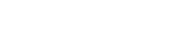= (–3, –4, –2)

Q (–3, –4, –2) is the hormonic conjugate of P (5, 4, – 6)

19. If (3, 2, – 1), (4, 1,1), and (6, 2, 5) are three vertices and (4, 2, 2) is the centroid of the tetrahedron, find the fourth Vertex.

Sol:

Given vertices of Tetrahedron are A (3, 2, – 1), B (4, 1,1), C (6, 2, 5) and centroid G = (4, 2, 2)

Let the fourth vertex is D = (x, y, z)

The centroid of the tetrahedron whose vertices are (x1, y1, z1), (x2, y2, z2) (x3, y3, z3), and (x4, y4, z4) is13 + x = 16, 5 + y = 8, 5 + z = 2

x = 3, y = 3, z = 3

∴ the fourth vertex D = (3, 3, 3)

20. Show that the points A(3, 2, –4), (5, 4, –6), and C(9, 8, –10) are collinear and find the ratio in which B divides AC.

Sol:

Given points are A(3, 2, –4), (5, 4, –6) and C(9, 8, –10)∴ points A (3, 2, –4), (5, 4, –6), and C (9, 8, –10) are collinear

B divides AB in the ratio is AB: BC =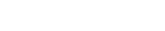##### Visit my Youtube Channel: Click on Below Logo# These notes cover all the topics covered in the intermediate First-year Maths syllabus and include plenty of solutions to help you solve all the major types of Math problems asked in the IPE examinations.

## Straight Lines

#### QUESTION1.

Prove that the points (1, 11), (2, 15), and (– 3, – 5) are collinear, and find the equation of the line containing them.

Sol:

Let A (1, 11), B (2, 15), and C (– 3, – 5)

The slope of the line segment joining the points (x1, y1) and (x2, y2) is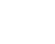QUESTION2.

Find the condition for the points (a, 0), (h, k), and (0, b) to be collinear.

Sol:

Let A (a, 0), B (h, k) and C (0, b)

The slope of the line segment joining the points (x1, y1) and (x2, y2) isGiven that A, B, and C are collinear points

The slope of AB = The slope of BC

⟹ – hk = (h – a) ( b – k)

– hk = hb – hk – ab + ak

⟹ 0 = hb + ak – ab

#### QUESTION3.

Find the equations of the straight lines which makes intercepts whose sum is sum is 5 and product is 6.

Sol:

Given that, a + b = 5 and ab = 6

⟹   b = 5 – a

a(5 – a) = 6

5a – a2 = 6

a2 – 5a + 6 = 0

a – 3a – 2a + 6 = 0

a (a – 3) – 2(a – 3) = 0

(a – 3) (a – 2) = 0

a = 3 or a = 2

case (i) if a = 3 ⟹ b = 2

case (ii) if a = 2 ⟹ b = 3

##### QUESTION4.

Find the equation of the straight line which makes an angle 1350 with the positive X – axis measured countered clockwise and passing through the point (– 2, 3).

Sol:

Slope of the line m = tan 1350 = – 1

The point is (– 2, 3)

The equation of the straight line in slope point form is (y – y1) = m (x – x1)

The equation of the line passing through the point (– 2, 3) with slope – 1 is

y – 3 = – 1 (x + 2)

y – 3 =– x – 2

x + y – 1 = 0

##### QUESTION5.

Find the equation of the straight line passing through the points (1, – 2) and (– 2, 3).

Sol:

Given points are (1, – 2), (– 2, 3)

The equation of the straight line in two points form is (y – y1) =(x – x1)

The equation of required straight line is

– 3 (y + 2) = 5 (x – 1)

– 3y – 6 = 5x – 5

5x + 3y + 1 = 0

QUESTION6.

Find the slopes of the line x + y = 0 and x – y = 0

Sol:

The slope of the line x + y = 0 is  = – 1

The slope of the line x – y = 0 is  = 1

#### QUESTION7.

Find the angle which the straight-line y =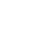x – 4 makes with the Y-axis.

Sol:

Compare with y = mx + c#### QUESTION8.

Find the equation of the reflection of the line x = 1 in the Y-axis.

Sol:

Given equation is     x = 1

Reflection about the Y-axis is x =– 1

Required equation of the line is x + 1 = 0

QUESTION9.

Write the equations of the straight lines parallel to X-axis and (i) at a distance of 3 units above the X-axis and (ii) at a distance of 4 units below the X-axis.

Sol:

(i) The equation of the straight line parallel to X-axis which is at a distance of 3 units above the X-axis is y = 3

⟹ y – 3 = 0

(ii) The equation of the straight line parallel to X-axis which is at a distance of 4   units below the X-axis is y = – 4

⟹ y + 4 = 0

QUESTION10.

Write the equations of the straight lines parallel to the Y-axis and (i) at a distance of 2 units from the Y-axis to the right of it (ii) at a distance of 5 units from the Y-axis to the left of it.

Sol:

(i) The equation of the straight line parallel to the Y-axis which is at a distance of 2 units from the Y-axis to the right of it is x = 2

⟹ x – 2 = 0

(ii) The equation of the straight line parallel to the Y-axis which is at a distance of 5 units from the Y-axis to the left of it is x =– 5

⟹ x + 5 = 0

#### QUESTION 11.

Find the value of x, if the slope of the line passing through (2, 5) and (x, 3) is 2.

Sol:

Given the slope of the line passing through (2, 5) and (x, 3) is 2.

The slope of the line segment joining the points (x1, y1) and (x2, y2) is⟹ 2(x – 2) = – 2

x – 2 = – 1

x = 1

#### QUESTION 12.

Find the equation of the straight line passing through (– 4, 5) and making non – zero intercepts on the coordinate axes whose sum is zero.

Sol:

Given a = b

### ⟹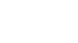x + y = a

but it is passing through (– 4, 5)

– 4+ 5 = a

a = 1

∴ The equation of the required straight line is x + y = 1

x + y – 1 = 0

QUESTION13.

Find the equation of the straight line passing through (– 2, 5) and cutting off equal and non – zero intercepts on the coordinate axes.

Sol:

Given a + b = 0

b = – a

x – y = a

but it is passing through (– 2, 4)

– 2– 4 = a

a = –6

∴ The equation of the required straight line is x – y = –6

x + y + 6 = 0

### Find the equation of the straight line whose distance from the origin is 4, if the normal ray from the origin to the straight line makes an angle of 1350 with  the positive direction of X – axis

Sol:

The equation of the straight line in the normal form is x cos α + y sin α = p

p = 4 and α = 1350

x cos 1350 + y sin 1350 = 4

QUESTION15.

### Write the parametric equation of the straight line passing through the point (3, 2) and making an angle 1350 with the positive direction of the X – axis.

Sol:

Given θ = 1350 and (h, k) = (3, 2)

The parametric equations are: x = h + r Cos θ, y = k +r Sin θ

x = 3 + r Cos 1350, y = 2 + r Sin 1350

### Transform the following equation into normal form.

(i)  x + y +1 = 0

Sol:

Given equation is x + y +1 = 0

x + y =– 1

– x – y = 1(ii) x + y = 2

Sol:

Given equation is x + y = 2### If the area of the triangle formed by the straight lines x = 0, y = 0 and 3x + 4y =a (a > 0) is 6 square units. Find the value of ‘a’.

Sol:

Given equation is 3x + 4y = a

The area of the triangle formed by the straight-line ax + by + c = 0 with the coordinate axes is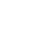a2 = 24 ⟹ a = 12(∵ a > 0)

QUESTION18.

Find the sum of the squares of the intercepts of the line 4x – 3y = 12

Sol:

Given equation is 4x – 3y = 12

a = 3 and b =– 4

the sum of the squares of intercepts = a2 + b 2

= 32 + (– 4)2

= 9 + 16 = 25

##### QUESTION 19.

Find the angle made by the straight – line y = – x + 3 with the positive direction of the X-axis measured in the counter-clockwise direction.

Sol:

It is in the form of y = mx + c

QUESTION 20.

Find the equation of the straight line in the symmetric form, given the slope and point on the line (2, 3).

Sol:

⟹ θ = 600

#### QUESTION 21.

If the product of the intercepts made by the straight-line x tan α + y sec α = 1 (0≤ α < π/2) on the coordinate axes is equal to sin α, find α.

Sol:

Given equation is x tan α + y sec α = 1

####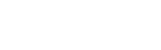a = cot α, b = cos α

product of intercepts = sin α

⟹ ab = sin α

cot α.cos α = sin α

(cos α)/ (sin α). cos α = sin α

⟹ cos2 α = sin2 α

⟹ tan2 α = 1

### tan α = 1(∵ 0≤ α < π/2)

∴ α = 450

QUESTION22.

If the sum of the reciprocals of the intercepts made by a variable straight–line on the axes of coordinates is a constant, then prove that the line always passes through a fixed point.

Sol:

Let the sum of the reciprocals of intercepts is k

⟹

∴ the line always passes through a fixed point (1/k, 1/k).

QUESTION23.

Find the ratio in which the straight-line 2x + 3y – 20 = 0 divides the join of the points (2, 3) and (2, 10).

Sol:

Given equation is L ≡ 2x + 3y – 20 = 0

L11 = 2(2) + 3(3) = 4 + 9– 20 = – 7

L22 = 2(2) + 3(10) = 4 + 30 – 20 = 14

We know that the line L = 0 divides the line joining the points (x1, y1) and (x2, y2) in the ratio – L11 : L22

= – (– 7) : 14

= 1 : 2

QUESTION24.

State whether (3, 2) (– 4, – 3) are on the same or opposite sides of the straight-line 2x – 3y + 4 = 0.

Sol:

Given equation is L ≡ 2x – 3y + 4 = 0

L11 = 2(3) – 3(2) + 4 = 6 – 6 + 4 = 4 > 0

L22 = 2(– 4) – 3(–3) + 4 = – 8 + 9 + 4 = 5 > 0

L11, L22 has the Same sign

∴ Given points lies the same side of the line 2x – 3y + 4 = 0.

QUESTION25.

Find the ratio in which (i) X-axis and (ii) Y-axis divide the line segment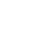joining A (2, –3) and B (3, – 6)

Sol:

(i) X – axis dividein the ratio – y1 : y2  = – ( –3) : – 6 = – 1 : 2

#### QUESTION26.

Find the equation of the straight line passing through the point of intersection of the lines x + y + 1 = 0 and 2x – y + 5 = 0 and containing the point (5, – 2).

Sol:

Given equations are

x + y + 1 = 0 ……… (1)

2x – y + 5 = 0……. (2)

The equation of the line passing through the point of intersection of the lines L1 = 0 and L2 = 0 is L1 + λ L2 = 0

The equation of the line passing through the point of intersection of the lines (1) and (2) is

x + y + 1 + λ (2x – y + 5) = 0

but the line passes through (5, – 2)

5 – 2 + 1 + λ (2(5) – (– 2) + 5) = 0

4 + λ (10 + 2 + 5) = 0

4 + λ (17) = 0

λ = –4 /17

∴ the equation of required line is

x + y + 1 + (–4 /17) (2x – y + 5) = 0

17(x + y + 1) –4 (2x – y + 5) = 0

17x + 17y + 17 – 8x + 4y – 20 = 0

9x + 21y – 3 = 0

3x + 7y – 1 = 0

QUESTION27.

If a, b, and c are in arithmetic progression, then show that the equation ax + by + c = 0 represents a family of concurrent lines and find the point of concurrency.

Sol:

Given, a, b, and c are in A.P. and the equation is ax + by + c = 0

a, b, and c are in A.P.

⟹ b = (a + c)/2

Substitute b value in the equation ax + by + c = 0

⟹  ax + [ (a + c)/2]y + c = 0

ax + ay/2 + cy/2 + c = 0

a(x + y/2) + c(y/2 + 1) = 0

dividing on both sides by a

⟹ (x + y/2) + (c/a) (y/2 + 1) = 0

It is in the form of L1 + λ L2 = 0

∴ given equation represent a family of concurrent lines

x + y/2 = 0 and y/2 + 1 = 0 are the lines

y/2 + 1 = 0 ⟹ y/2 = – 1

y =– 2 ⟹ x = 1

The point of concurrency is (– 2, 1)

QUESTION28.

Find the point of intersection of the line 7x + y + 3 = 0 and x + y = 0

Sol:

Given equations are 7x + y + 3 = 0 and x + y = 0#### QUESTION29.

Transform the following equations into the form L1 + λ L2 = 0 and find the point of concurrency of the family of straight lines represented by the equation.

(i) (2 + 5k) x – 3 (1 + 2k) y + (2 – k) = 0

Given equation is

(2 + 5k) x – 3 (1 + 2k) y + (2 – k) = 0

2x + 5kx – 3y – 6ky + 2 – k = 0

2x – 3y + 2 + k (5x – 6y – 1) = 0

It is in the form of L1 + λ L2 = 0 L1 ≡ 2x – 3y + 2 = 0 and L2≡ 5x – 6y – 1 = 0

By solving above equations, we get point of concurrency∴ The point of concurrency is (5, 4)

(ii) ( k + 1) x + (k + 2) y + 5 = 0

Given equation is  (k + 1) x + (k + 2) y + 5 = 0

kx + x + ky + 2y + 5 = 0

x + 2y + 5 + k (x + y) = 0

It is in the form of L1 + λ L2 = 0

L1 ≡ x + 2y + 5 = 0 and L2≡ x + y = 0

By solving the above equations, we get point of concurrency∴ The point of concurrency is (5, – 5)

QUESTION30.

Find the value of ‘p’, if the straight lines x + p = 0, y + 2 = 0 and 3x + 2y + 5 = 0 are concurrent.

Sol:

Given that x + p = 0 —— (1), y + 2 = 0 —— (2) and 3x + 2y + 5 = 0 —— (3)   are concurrent.

From (1) x + p = 0 ⟹ x = – p

From (2) y + 2 = 0 ⟹ y = – 2

Point of intersection of (1) and (2) is (– p, – 2)

From (3)

3y + 2x + 5 = 0

3(– p) + 2 (– 2) + 5 = 0

– 3p – 4 + 5 = 0

– 3p + 1 = 0

3p = 1

p = 1/3

##### QUESTION31.

Find the area of the triangle formed by the following straight lines and the coordinate axes.

(i) x – 4y + 2 = 0

The area of the triangle formed by the straight-line ax + by + c = 0 with the coordinate axes isThe area of the triangle formed by the straight-line x – 4y + 2 = 0

(ii) 3x – 4y + 12 = 0

The area of the triangle formed by the straight-line 3x – 4y + 12 = 0 with the coordinate axes is  =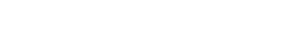QUESTION32.

Find the angle between the lines 2x + y + 4 = 0 and y – 3x =7

Sol:

Given Equations are 2x + y + 4 = 0 and y – 3x =7

If θ is the angle between the lines a1x + b1y + c = 0 and a2x + b2y + c = 0, then

∴ θ = 450

QUESTION33.

Find the perpendicular distance from the point (– 3, 4) to the straight line 5x – 12y = 2

Sol:

Given equation is 5x – 12y = 2 ⟹ 5x – 12y – 2 = 0

The perpendicular distance from the point (x1, y1) to the straight-line ax + by + c = 0 is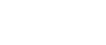The perpendicular distance from the point (– 3, 4) to the straight-line 5x – 12y – 2 = 0 is

= 5

QUESTION34.

Find the distance between the parallel lines 3x + 4y – 3 = 0 and 6x + 8y – 1 = 0

Sol:

Given equations are 3x + 4y – 3 = 0 and 6x + 8y – 1 = 0

3x + 4y – 3 = 0 ⟹ 2(3x + 4y – 3) = 2(0) (multiplying on both sides by 2)

⟹ 6x + 8y – 6 = 0

The distance between two parallel lines ax + by + c1 =0 and ax + by + c2 =0 is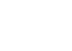The distance between two parallel lines 6x + 8y – 6 = 0 and 6x + 8y – 1 = 0 is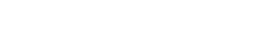#### QUESTION35.

Find the foot of the perpendicular from (– 1, 3) on the straight line 5x – y – 18 = 0

Sol:

Let (h, k) be the foot of the perpendicular from (– 1, 3) on the straight line 5x – y – 18 = 0

If (h, k) is the foot of the perpendicular from (x1, y1) on the straight-line ax + by + c = 0, then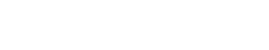h+ 1 = 5 and k – 3 = – 1

h = 4 and k = 2

∴ (h, k) = (4, 2)

QUESTION36.

Find the image of (1, – 2) with respect to the straight line 2x – 3y + 5 = 0

Sol:

Let  (h, k) be the image of (1, – 2) with respect to the straight line 2x – 3y + 5 = 0

If (h, k) is the image of the point (x1, y1) with respect to the straight line ax + by + c = 0, then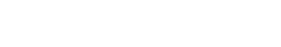If (h, k) is the image of (1, – 2) with respect to the straight line 2x – 3y + 5 = 0,

h – 1 = – 4 and k + 2 = 6

h =– 3 and k = 4

∴ (h, k) = (– 3, 4)

QUESTION37.

If 2x – 3y – 5 = 0 is the perpendicular bisector of the line segment joining (3, –4) and (α, β), find α + β.

Sol:

Given 2x – 3y – 5 = 0 is the perpendicular bisector of the line segment joining (3, –4) and (α, β)

⟹ (α, β) is the image of (3, –4) concerning the straight line 2x – 3y – 5 = 0

α – 3 = – 4 and β + 4 = 6

α = – 1 and β = 2

α + β = – 1 + 2 = 1

QUESTION38.

Find the incenter of the triangle whose vertices are (1, ), (2, 0) and (0, 0)

Sol:in centre of a triangle with sides a, b and c, whose vertices (x1, y1), (x2, y2) and (x3, y3) is

QUESTION39.

Find the ortho centre of the triangle whose sides are given by x + y + 10 = 0, x – y – 2 = 0 and 2x + y – 7 = 0.

Sol:

Given equations are

x + y + 10 = 0 …… (1)

x – y – 2 = 0 …… (2)

2x + y – 7 = 0. …… (3)

Slope of the line (1) is – 1

Slope of the line (2) is 1

The straight lines (1) and (2) are perpendicular lines

The point of intersection of (1) and (2) is the orthocenter

x + y = – 10 ⟹ y = – 10 – x

from (2) x – y – 2 = 0

x – (– 10 – x) – 2 = 0

x + 10 + x – 2 = 0

2x + 8 = 0 ⟹ x = – 4

⟹ y = – 10 + 4 = – 6

∴ Orthocenter is (– 4, – 6)

QUESTION40.

Find the circum centre of the triangle whose sides are x = 1, y = 1 and x + y = 1

Sol:

Given equations are x = 1, y = 1 and x + y = 1A = (1, 0), B = (1, 1) and C = (0, 1)

AB and BC are perpendicular lines

Circum centre is the midpoint of hypotenuse

i.e., midpoint of AC

###### Functions (2M Questions &Solutions)|| V.S.A.Q.’S||

Functions (2M Questions &Solutions): This note is designed by the ‘Basics in Maths’ team. These notes to do help intermediate First-year Maths students.

Inter Maths – 1A two marks questions and solutions are very useful in IPE examinations.

These notes cover all the topics covered in the intermediate First-year Maths syllabus and include plenty of solutions to help you solve all the major types of Math problems asked in the

IPE examinations.

### Functions

QUESTION 1

Find the Domain of the following real-valued functions.

It is defined when 6x – x2 – 5 ≠ 0

⇒ x2 – 6x + 5 ≠ 0

x2 – 5x – x + 5 ≠ 0

x (x – 5) –1(x – 5) ≠ 0

(x – 5) (x – 1) ≠ 0

x ≠ 5 or x ≠ 1

∴ domain = R – {1, 5}

It is defined when 3 + x ≥ 0, 3 – x ≥ 0 and x ≠ 0

⇒ x ≥ –3, x ≤ 3 and x ≠ 0

⇒   –3≤ x, x ≤ 3 and x ≠ 0

⇒   –3≤ x ≤ 3 and x ≠ 0

x ∈ [–3, 3] – {0}

∴ domain = [–3, 3] – {0}

It is defined when x + 2 ≥ 0, 1 – x > 0 and 1 – x ≠ 0

⇒ x ≥ –2, x < 1 and x ≠ 0

x ∈ [–2, ∞) ∩ (– ∞, 1) – {0}

⇒ x ∈ [–2, 1) – {0}

∴ domain = [–2, 1) – {0}

It is defined when 4x – x2 ≥ 0

⇒ x2 – 4x ≤ 0

x (x – 4) ≤ 0

(x – 0) (x – 4) ≤ 0

x ∈ [0, 4]

∴ domain = [0, 4]

(v) f(x) = log (x2 – 4x + 3)

Given f(x) = log (x2 – 4x + 3)

It is defined when x2 – 4x + 3 > 0

⇒   x2 – 3x – x + 3 > 0

x (x – 3) –1(x – 3) > 0

(x – 3) (x – 1) > 0

x ∈ (–∞, 1) ∪ (3, ∞)

x ∈ R – [1, 3]

∴ domain = R – [1, 3]

(x + 1)(x – 1) ≥ 0 and x2 – 2x – x + 2 > 0

(x + 1) (x – 1) ≥ 0 and x (x – 2) (x – 1) > 0

x∈ (–∞, –1) ∪ (1, ∞) and x ∈ (–∞, –1) ∪ (2, ∞)

∴ domain = R – (–1, 2]

⇒ x < 0

∴ domain = (–∞, 0)

⇒ x > 0

∴ domain = (0, ∞)

QUESTION 2

If f : R→ R , g : R → R defined by f (x ) = 4x – 1, g(x) = x2 + 2 then find (i) (gof) (x) (ii) (gof) (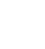)  (iii) (fof) (x)  (iv) go(fof) (0).

Sol: Given f(x) = 4x – 1, g(x) = x2 + 2

(i)  (gof) (x) = g (f (x))

= g (4x – 1)

= (4x – 1)2 + 2

= 16x2 – 8x + 1 + 2

= 16x2 – 8x + 3

= g (a + 1 – 1)

= g(a)

= a2 + 2

(iii) (fof) (x) = f (f (x))

= f (4x – 1)

= 4 (4x – 1) – 1

= 16x – 4 – 1

= 16x – 5

(iv) go(fof) (0) = g(fof) (0)

= g (f (f (0)))

= g (f (– 1))

= g (– 4 – 1)

= g (– 5)

= (– 5)2 + 2

= 25 + 2 = 27

QUESTION 3

If f and g are real valued functions defined by f(x) = 2x – 1 and g(x) = x2, then find (i) (3f – 2g) (x) (ii) (fg)(x) (iii)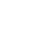(x) (iv) (f + g+ 2) (x)

Sol: Given f(x) = 2x – 1 and g(x) = x2

(i) (3f – 2g) (x) = 3f(x) – 2 g(x)

= 3(2x – 1) – 2(x2)

= 6x – 3 – 2x2

(ii) (fg)(x) = f(x) g(x)

= (2x – 1) (x2)

= 2x3 – 3x2

(iv) (f + g+ 2) (x) = f(x) + g(x) + 2

= 2x – 1 + x2 + 2

= x2 + 2x  + 1

QUESTION 4

If f = {(4, 5), (5, 6), (6, – 4)} g = {(4, – 4), (6, 5), (8,5)}, then find (i) f + g (ii) f – g (iii) 2f +4g (iv) f + 4 (v) fg (vi) f/g (vii)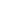(viii)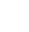(ix) f2 (x) f3

Sol: Given f = {(4, 5), (5, 6), (6, – 4)}

g = {(4, – 4), (6, 5), (8,5)}

The domain of f ∩ The Domain of g = {4, 6}

(i) (f + g) (4) = f (4) + g (4)

= 5 – 4 = 1

(f + g) (6) = f (6) + g (6)

=– 4 + 5 = 1

∴ f + g = {(4, 1), (6, 1)}

(ii) (f – g) (4) = f (4) – g (4)

= 5 – (– 4) = 5 + 4 = 9

(f – g) (6) = f (6) – g (6)

= – 4– 5   = – 9

∴ f – g   = {(4, 9), (6, – 9}

(iii) (2f +4g) (4) = 2 f (4) + 2 g (4)

= 2(5) + 4 (– 4)

= 10 – 16

=– 6

(2f +4g) (6) = 2 f (6) + 2 g (6)

= 2(– 4) + 4 (5)

= – 8 + 20

=12

∴ (2f +4g) = {(4, – 6), (6, 12)}

(iv) (f + 4) (4) = f (4) + 4 = 5 + 4 = 9

(f + 4) (5) = f (5) + 4 = 6 + 4 = 10

(f + 4) (6) = f (6) + 4 = – 4 + 4 = 0

∴ (f + 4) = {(4, 9), (5, 10), (6, 0)}

(v) fg (4) = f (4) g (4) = (5) (– 4) =– 20

fg (6) = f (6) g (6) = (– 4) (5) =– 20

∴ fg = {(4, – 20), (6, – 20)}

(vi) f/g (4) = f(4)/g(4) = 5/ – 4 = – 5/4

f/g (6) = f(6)/g(6) = – 4/ 5

∴ f/g = {(4, – 5/4), (6, – 4/ 5)}(ix) f2(4) = (f (4))2 = (5)2 = 25

f2(5) = (f (5))2 = (6)2 = 36

f2(6) = (f (6))2 = (– 4)2 = 16

∴ f2 = {(4, 25), (5, 36), (6, 16)}

(x) f3(4) = (f (4))3 = (5)3 = 125

f3(5) = (f (5))3 = (6)3 = 216

f3(6) = (f (6))3 = (– 4)3 = –64

∴ f3 = {(4, 125), (5, 216), (6, –64)}

QUESTION 5

If A = {0, π/6, π/4, π/3, π/2} and f: A→ B is a surjection defined by f(x) = cos x, then find B.

Sol: Given A = {0, π/6, π/4, π/3, π/2}

f(x) = cos x

f (0) = cos (0) = 1

f(π/3) = cos (π/3) = 1/2

f(π/2) = cos (π/2) = 0

QUESTION 6

If A = {–2, –1, 0, 1, 2} and f: A→ B is a surjection defined by f(x) =x2 + x + 1, then find B.

Sol: Given A = {–2, –1, 0, 1, 2} and f(x) = x2 + x + 1

f (–2) = (–2)2 + (–2) + 1= 4 – 2 + 1 = 3

f (–1) = (–1)2 + (–1) + 1= 1 – 1 + 1 = 1

f (0) = (0)2 + (0) + 1= 0 + 0 + 1 = 1

f (1) = (1)2 + (1) + 1= 1 + 1 + 1 = 3

f (2) = (2)2 + (2) + 1= 4 + 2 + 1 = 7

∴ B = {1, 3, 7}

QUESTION 7

If A = {1, 2, 3, 4} and f: A→ B is a surjection defined by f(x) =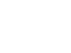then find B.

QUESTION 8

If f(x) = 2, g(x) = x2, h(x) = 2x ∀ x ∈ R, then find (fo(goh)) (x)

Sol: Given f(x) = 2, g(x) = x2, h(x) = 2x

(fo(goh)) (x) = fo (g (h (x))

= f (g (h (x))

= f(g(2x)

= f((2x)2)

= f(4x2) = 2

QUESTION 9

If f: R→ R, g: R → R defined by f (x) = 3x – 2, g(x) = x2 +1 then find (i) (gof-1) (2) (ii) (gof) (x – 1)

Sol: Given f: R→ R, g: R → R defined by f (x) = 3x – 2, g(x) = x2 +1

(i) Let y = f(x) ⟹ x = f-1(y)

y = 3x – 2

y + 2 = 3x

x = (y + 2)/3

f-1(y) = (y + 2)/3

∴ f-1(x) = (x + 2)/3

Now

(gof-1) (2) = g(f-1(2))

= g ((2 + 2)/3)

= g (4/3)

= (4/3)2 + 1 = 16/9 + 1 = 25/9

(ii) (gof) (x – 1) = g (f (x – 1))

= g [ 3(x – 1) – 2)]

= g (3x – 3 – 2)

=g (3x – 5)

= (3x – 5)2 + 1

= 9x2 – 30x + 25 + 1

= 9x2 – 30x + 26

QUESTION 10

If f: N→ N defined by f (x) = 2x + 5, Is onto? Explain with reason.

Sol: Given f (x) = 2x + 5

Let y = f(x) ⟹ x = f-1(y)

y = 2x + 5

2x = y – 5

x = (y – 5)/2 ∉ N

∴ f(x) is not onto

QUESTION 11

Find the inverse of the following functions

(i) If a, b ∈ R, f: R→ R defined by f(x) = ax + b

Given function is f(x) = ax + b

Let y = f(x) ⟹ x = f-1(y)

y = ax + b

y – b = ax

x = (y – b)/a

f-1(y) = (y – b)/a

∴ f-1(x) = (x – b)/a

(ii) f: R→ (0, ∞) defined by f(x) = 5x

Given function is f(x) = 5x

Let y = f(x) ⟹ x = f-1(y)

y = 5x

Let y = f(x) ⟹ x = f-1(y)

x = 2y

f-1(y) = 2y

∴ f-1(x) = 2x

(iv) f: R→ R defined by f(x) = e4x + 7

Given function is f(x) = e4x + 7

Let y = f(x) ⟹ x = f-1(y)

y = e4x + 7

(v) f: R→ R defined by f(x) = (2x + 1)/3

Given function is f(x) = (2x + 1)/3

Let y = f(x) ⟹ x = f-1(y)

y= (2x + 1)/3

3y = 2x + 1

2x = 3y – 1

x = (3y – 1)/2

f-1(y) = (3y – 1)/2

∴ f-1(x) = (3x – 1)/2

QUESTION 12

If f: R→ R defined by f(x) =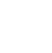, then show that f (tan θ) = cos 2θ

∴  f (tan θ) = cos 2θ

QUESTION 13

QUESTION 14

If the function f: R→ R defined by f(x) =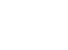, then show that f (x + y) + f (x – y) = 2 f(x) f(y).

∴ f (x + y) + f (x – y) = 2 f(x) f(y)

QUESTION 15

Sol: Given function is f(x) = cos (log x)= cos (log x) cos (log y) – cos (log x) cos (log y)

= 0

Hence proved

QUESTION 16

Find the range of the following real-valued functions

Sol:

ey > 0

∴ The range of f(x) is R

It is defined when x – 2 ≠ 0

⟹ x ≠ 2

Domain = R – {2}

y = x + 2

if x = 2 ⟹ y = 4

∴ Range of f(x) is R – {4}

QUESTION 17

Find the domain and range of the following real-valued functions

Sol:

It is defined when 1 + x2 ≠ 0

⟹ x2 ≠ – 1

x ∈ R

∴ domain of f(x) is R

y (1 + x2) = x

y + x2y = x

x2 y – x + y = 0

It is defined when 1 – 4y2 ≥ 0 and 2y ≠ 0

⟹ 4y2 – 1 ≤ 0 and y ≠ 0

(2y – 1) (2y + 1) ≤ 0 and y ≠ 0

(y – 1/2) (y + 1/2) ≤ 0 and y ≠ 0

–  ½≤ y ≤ ½ and y ≠ 0

∴ Range of f(x) is [– ½, ½] – {0}

It is defined when 9 – x2 ≥ 0

⟹ x2 – 9 ≤ 0

(x + 3) (x – 3) ≤ 0

–  3 ≤ y ≤ 3

∴ the domain of f (x) is [– 3, 3]

y2 = 9 – x2

x2 = 9 – y2

It is defined when 9 – y2 ≥ 0

⟹ y2 – 9 ≤ 0

(y + 3) (y – 3) ≤ 0

–  3 ≤ y ≤ 3

y ∈ [– 3, 3]

∴ Range of f (x) is [0, 3] (∵ y ≥ 0)

clearly, x ∈ R

∴ domain of f(x) is R

If x = 0, then y = 1

If x = – 1, then y = 1

If x = 1, then y = 3

If x = – 2, then y = 3

If x = 2, then y = 5

∴ Range of f (x) is [1, ∞)

(iv) f(x) = [x]

clearly Domain = R  and Range = Z

QUESTION 18.

Define (i) One – One function (ii) Onto function (iii) Bijection (iv) Even and Odd functions

(i) One – One Function: one – one, if every element of A has a unique image in B.

(ii) Onto function: A function f: A→ B is said to be onto if ∀ y ∈ B there exists x ∈ A such that f(x) = y.

(iii) Bijection: A function f: A→ B is said to be a Bijection if it is both one-one and onto.

(iv) Even and Odd functions:

If f(–x) = f(x), then f(x) is even function

If f(– x)

= – f(x), then f(x) is odd function

##### Visit my Youtube Channel: Click on Below Logo